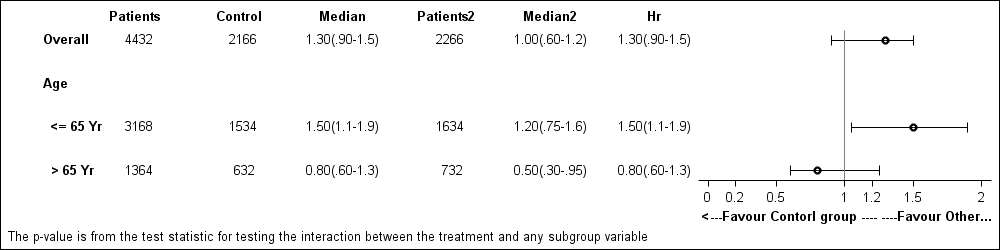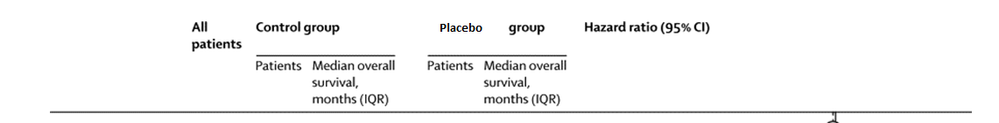## proc template

HI All,

I am working on per-processed data under proc template for forest plot. I am using sas9.2.

i attached the file which i am working for the layout.

Thanks

Sam17 REPLIES 17

## Re: proc template

Hi All,

My template code is as follows, not working properly

proc template;

define statgraph Forest;

dynamic _show_bands;

begingraph /designwidth=1000px designheight=600px;;

entrytitle halign=left "Company Name" halign=right "Study Phase"/ pad=(bottom=5px);

entrytitle halign=left "Drug Name" halign=right "Detailed Study information"/pad=(bottom=5px);

entrytitle "Duration of Survival by Overall Survival Status"/ pad=(bottom=5px);

entrytitle halign=left "Treatment Group I Treatment Group II"/pad=(bottom=0px);

layout lattice / columns=8 columngutter=0 columnweights=(0.21 0.09 0.4 0.15 0.4 0.15 0.15 0.35);

sidebar / align=top;

layout lattice / rows=2 columns=8 columnweights=(0.21 0.09 0.4 0.15 0.4 0.15 0.15 0.35);

entry textattrs=(size=8) halign=left "Baseline Risk Factor";

entry textattrs=(size=8) " Total";

entry " Event";

entry textattrs=(size=8) "Median (95%CI) mo";

entry " Event";

entry halign=right textattrs=(size=8) " Median (95%CI) mo " ;

entry halign=right textattrs=(size=8) "Hazard Ratio(95%)" ;

entry " ";

entry " ";

entry " ";

entry halign=right textattrs=(size=6) "_________________________________";

entry halign=right textattrs=(size=6) "_________________________________";

entry halign=right textattrs=(size=6) "_________________________________";

entry halign=right textattrs=(size=6) "_________________________________";

entry halign=right textattrs=(size=6) " ";

entry " ";

endlayout;

endsidebar;

and in log:

NOTE: COLUMNWEIGHTS=(0.21 0.09 0.4 0.15 0.4 0.15 0.15 0.35) is invalid. The default will be used.

NOTE: COLUMNWEIGHTS=(0.21 0.09 0.4 0.15 0.4 0.15 0.15 0.35) is invalid. The default will be used.

## Re: proc template

35143 - Forest plot has an example of creating a forest plot.

What do you mean "not working properly"? Error messages? No output? Unexpected output?

## Re: proc template

Thank you ballardw,

I adopted from the above mentioned paper.. but my layout is bit different that where i struck

Thanks

Sam

## Re: proc template

Hi Ballardw,

Working!!! no error message on the log, but output is not reflecting the layout what i required(attached)

and in log:

NOTE: COLUMNWEIGHTS=(0.21 0.09 0.4 0.15 0.4 0.15 0.15 0.35) is invalid. The default will be used.

NOTE: COLUMNWEIGHTS=(0.21 0.09 0.4 0.15 0.4 0.15 0.15 0.35) is invalid. The default will be used.

Thanks

SamJay54
Meteorite | Level 14

## Re: proc template

At SAS 9.2, columns weights should add up to 1.0.  For later releases, the sum of the weights is normalized automatically.

There are many examples on Graphically Speaking for Forest plots, both SAS 9.3 and 9.2:

http://blogs.sas.com/content/graphicallyspeaking/2013/01/06/forest-plot-with-sas-9-3/

It is easier to help if you attach the full working code with data.

## Re: proc template

Thank you Sanjay!!!

I am working on one your forest plot example. i am just trying to achieve different layout which i attached in my first post.

i got unexpected plot,

the following is sample data and code

data:

data test;

informat subgroup \$25.;

input Subgroup & allpat Count med &\$13 mean low high _count _med &\$13 _mean _low _high hr &\$13;

datalines;

Overall  4432 2166 1.30(.90-1.5) 1.3 0.90 1.50 2266 1.00(.60-1.2) 1.0 0.60 1.20 1.30(.90-1.5)

Age         .    .                .   .    .      .                .   .    .

<= 65 Yr 3168 1534 1.50(1.1-1.9) 1.5 1.05 1.90 1634 1.20(.75-1.6) 1.2 0.75 1.60 1.50(1.1-1.9)

> 65 Yr  1364  632 0.80(.60-1.3) 0.8 0.60 1.25  732 0.50(.30-.95) 0.5 0.30 0.95 0.80(.60-1.3)

;

run;

proc template;

define statgraph Forest;

dynamic _show_bands;

begingraph /designwidth=1000px designheight=600px;;

layout lattice / columns=8 columngutter=0 columnweights=(0.21 0.09 0.4 0.15 0.4 0.15 0.15 0.35);

sidebar / align=top;

layout lattice / rows=2 columns=8 columnweights=(0.21 0.09 0.4 0.15 0.4 0.15 0.15 0.35);

entry textattrs=(size=8) halign=left "Subgroup";

entry textattrs=(size=8) " All Patients";

entry " ";

entry textattrs=(size=8) "Control group";

entry " ";

entry halign=right textattrs=(size=8) "Other group" ;

entry halign=right textattrs=(size=8) "Hazard Ratio(95%)" ;

entry " ";

entry " ";

entry " ";

entry halign=right textattrs=(size=6) "_________________________________";

entry halign=right textattrs=(size=6) "_________________________________";

entry halign=right textattrs=(size=6) "_________________________________";

entry halign=right textattrs=(size=6) "_________________________________";

entry halign=right textattrs=(size=6) " ";

entry " ";

endlayout;

endsidebar;

/*--First Subgroup column, shows only the Y2 axis--*/

layout overlay / walldisplay=none xaxisopts=(display=none)

y2axisopts=(reverse=true display=(tickvalues)

tickvalueattrs=(weight=bold));

scatterplot y=subgroup x=zero / markerattrs=(size=0) yaxis=y2;

endlayout;

/*--Second column showing Count and percent--*/

layout overlay / xaxisopts=(display=none)

y2axisopts=(reverse=true display=none) walldisplay=none;

if (exists(_show_bands))

referenceline y=ref / yaxis=y2 lineattrs=(thickness=15 color=cxf0f0f0);

endif;

scatterplot y=subgroup x=zero / markercharacter=allpat yaxis=y2

markercharacterattrs=graphvaluetext;

endlayout;

/*--3rd column showing PCIGroup and Group columns--*/

layout overlay / x2axisopts=(display=(tickvalues) offsetmin=0.25 offsetmax=0.25)

y2axisopts=(reverse=true display=none) walldisplay=none;

if (exists(_show_bands))

referenceline y=ref / yaxis=y2 lineattrs=(thickness=15 color=cxf0f0f0);

endif;

scatterplot y=subgroup x=pat_lbl / markercharacter=count xaxis=x2 yaxis=y2

markercharacterattrs=graphvaluetext;

endlayout;

/*--4th column showing PCIGroup and Group columns--*/

layout overlay / x2axisopts=(display=(tickvalues) offsetmin=0.25 offsetmax=0.25)

y2axisopts=(reverse=true display=none) walldisplay=none;

if (exists(_show_bands))

referenceline y=ref / yaxis=y2 lineattrs=(thickness=15 color=cxf0f0f0);

endif;

scatterplot y=subgroup x=med_lbl / markercharacter=med xaxis=x2 yaxis=y2

markercharacterattrs=graphvaluetext;

endlayout;

/*--5th/6th column showing PCIGroup and Group columns--*/

layout overlay / x2axisopts=(display=(tickvalues) offsetmin=0.25 offsetmax=0.25)

y2axisopts=(reverse=true display=none) walldisplay=none;

if (exists(_show_bands))

referenceline y=ref / yaxis=y2 lineattrs=(thickness=15 color=cxf0f0f0);

endif;

scatterplot y=subgroup x=pat_lbl / markercharacter=_count xaxis=x2 yaxis=y2

markercharacterattrs=graphvaluetext;

endlayout;

/*--5th/6th column showing PCIGroup and Group columns--*/

layout overlay / x2axisopts=(display=(tickvalues) offsetmin=0.25 offsetmax=0.25)

y2axisopts=(reverse=true display=none) walldisplay=none;

if (exists(_show_bands))

referenceline y=ref / yaxis=y2 lineattrs=(thickness=15 color=cxf0f0f0);

endif;

scatterplot y=subgroup x=med_lbl / markercharacter=_med xaxis=x2 yaxis=y2

markercharacterattrs=graphvaluetext;

endlayout;

/*--7th column showing PCIGroup and Group columns--*/

layout overlay / x2axisopts=(display=(tickvalues) offsetmin=0.25 offsetmax=0.25)

y2axisopts=(reverse=true display=none) walldisplay=none;

if (exists(_show_bands))

referenceline y=ref / yaxis=y2 lineattrs=(thickness=15 color=cxf0f0f0);

endif;

scatterplot y=subgroup x= zero/ markercharacter=hr xaxis=x2 yaxis=y2

markercharacterattrs=graphvaluetext;

endlayout;

/*--Third column showing odds ratio graph--*/

layout overlay / xaxisopts=(label=' <---Favour Contorl group ---- ----Favour Other group--->'

linearopts=(tickvaluepriority=true

tickvaluelist=(0.0 0.2 0.5 1.0 1.2 1.5 2.0)))

y2axisopts=(reverse=true display=none) walldisplay=none;

if (exists(_show_bands))

referenceline y=ref / yaxis=y2 lineattrs=(thickness=15 color=cxf0f0f0);

endif;

scatterplot y=subgroup x=mean / xerrorlower=low xerrorupper=high yaxis=y2

markerattrs=(symbol=circle);

*scatterplot y=subgroup x=_mean / xerrorlower=_low xerrorupper=_high yaxis=y2

markerattrs=(symbol=circle);

referenceline x=1/ lineattrs=(pattern=solid);;

endlayout;

endlayout;

entryfootnote halign=left textattrs=(size=7)

'The p-value is from the test statistic for testing the interaction between the '

'treatment and any subgroup variable';

endgraph;

end;

run;

proc template;

define style listingSF;

parent = Styles.Listing;

style GraphFonts from GraphFonts

"Fonts used in graph styles" /

'GraphDataFont' = ("<sans-serif>, <MTsans-serif>",7pt)

'GraphValueFont' = ("<sans-serif>, <MTsans-serif>",7pt)

'GraphLabelFont' = ("<sans-serif>, <MTsans-serif>",7pt, bold);

;

end;

run;

ods graphics / reset width=7in height=5in imagename='Forest_Ref_92';

proc sgrender data=test template=Forest;

dynamic _show_bands='YES';

run;

Thanks

samJay54
Meteorite | Level 14

## Re: proc template

Please attach your original SAS program file.  Cutting and pasting from here is messing up the input section of your code.Jay54
Meteorite | Level 14

## Re: proc template

There are a few issues with your code.  I had to simplify the input statement as your inline formatting with the & modifiers were not working.  I simplified the template so all the required values are displayed in 3 columns, one for the Subgroups, one for the values and one for the odds ratio graph.  You can add back the header as appropriate.## Re: proc template

Hi Sanjay,

Thank so much for your time. I tried with my values but i got hard to fit in it. I dont know how to get the label part fix(control group ,placebo group)

find the sample code , data and layout style.

Thanks

SamJay54
Meteorite | Level 14

## Re: proc template

The header is separately customized in the SideBar.  You will have to size the header cells as you need to get the headers in the right places.

## Re: proc template

Hi Sanjay,

i am exploring more on forest plot. I am considering few examples from your forum

http://blogs.sas.com/content/graphicallyspeaking/?s=forest+plot

Example :Figure 12.3:  SAS 9.3 Forest Plot:

i would like to achieve this kind of plot by using proc template , your example using proc sgplot

i am stumped with it!!!

any help is greatly helpful for learner like me !!!

Thanks

SamJay54
Meteorite | Level 14

## Re: proc template

You will need to provide a bit more information on what aspect you are stumped with.  Note: A link to the full program for the graph is provided at the bottom of the article.  The program already uses GTL and not SGPLOT.

## Re: proc template

Hi Sanjay,

Thank you for your quick response !!!

http://blogs.sas.com/content/graphicallyspeaking/files/2012/02/Full-SAS-93-Code.txt

this code is with GTL and proc sgplot

But i would like to achieve the same plot with

GTL+ proc sgrender

2)And for Overall , how do we get the symbol=diamondfilled?

Thanks

SamJay54
Meteorite | Level 14

## Re: proc template

Discussion stats
• 17 replies
• 3399 views
• 0 likes
• 3 in conversation